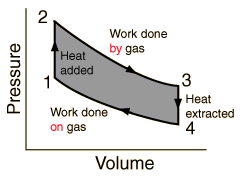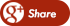﻿ WHET IS THE RELATION BETWEEN HEAT VS WORK?Back

## WHET IS THE RELATION BETWEEN HEAT VS WORK?

By Admin onPerhaps the most interesting property of thermal energy is that it spontaneously flows from one object to another, provided that the first object is hotter than the second. That’s why ice melts in a warm room, fingers freeze on cold metal, and sunlight continuously warms the cool earth.

When energy spontaneously flows from a hotter object to a cooler object, we refer to that energy (while in transit) as heat. Heat is to be contrasted with work, which is any other type of energy transfer (such as exerting a force through a distance, as discussed in the previous chapter).

This use of the word “heat” takes quite a bit of getting used to. For instance, we should never say that a cup of boiling water “contains lots of heat,” because once the energy is in the water, it doesn’t matter whether the energy entered as heat or as work. If you put the water over a stovetop, then energy enters as heat. But you could vigorously shake or stir the water, with the same end result (hot water), accomplished this time by performing mechanical work. Or you could put the cup of water into a microwave oven: Since the flow of microwave energy from the magnetron source into the water is not caused by the magnetron being hotter than the water, we should classify this energy transfer as work (in this case, electrical work), not as heat.

Any transfer of energy from one object to another can be classified as either heat or work. This means that whenever the energy content of an object changes, that change must have been caused by heat flow, or work, or some combination of the two. For instance, if you add 2 MJ of heat to a pot of water and simultaneously perform 1 MJ of work, then the water’s energy must increase by 3 MJ.In general, change in energy = (heat added) + (work done), or in terms of symbols,

∆E = H + W,

where

E is the total energy content of an object,

H is the amount of heat added to it, and

W is the amount of work done on it (during some time period of interest).

If heat or work is extracted from the object, then we simply use a negative value for H or W, and the same equation applies.